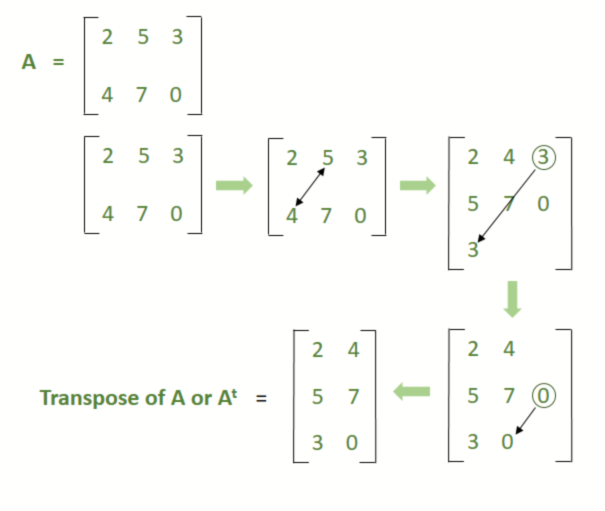Transpose of a matrix – Matrices | Class 12 Maths

• Last Updated : 09 Nov, 2020

The knowledge of matrices is necessary for various branches of mathematics. Matrices are one of the most powerful tools in mathematics. Now see one of the features of the matrix in this article.

Transpose of a matrix

This one of the main properties of the matrix. The meaning of transpose is to exchange places of two or more things. In the case of the matrix, transpose meaning changes the index of the elements. In this case, we swap the row-element with the column-element or vise versa.

Let, A is a matrix of size m × n and At is the transpose of matrix A,
where [a(ij)] of A = [a(ji)] of At, here 1 ≤ i ≤ m and 1 ≤ j ≤ n

Example:

Let, the size of matrix A is 2 × 3,Therefore,

Transpose of A orAfter transpose it becomes 3 × 2Properties of Transpose

Transpose of Product of Matrices

This property says that, (AB)t = BtAt

Proof

Here A and B are two matrices of size m × n and n × p respectively

and At and Bt are their transpose form of size n × m and p × n respectively (from the product rule of matrices).

It implies, if A = [a(ij)], and At = [c(ji)]

Then, [c(ji)] = [a(ij)]

and,

If B = [b(jk)], and Bt = [d(kj)]

Then, [d(kj)] = [b(jk)]

Now, from the product rule of matrices we can write,

AB is m × p matrix and (AB)t is p × m matrix.

Also, Bt is a p × n matrix and At is a n × m matrix.

This implies that,

(Bt)(At) is a p × m matrix.

Therefore,

(AB)t and (Bt)(At) are both p × m matrices.

Now we can write,

(k, i)th element of (AB)t = (i, k)th element of AB(k, i)th element of (Bt)(At)

Therefore,

the elements of (AB)t and (Bt)(At) are equal.

Therefore,

(AB)t = (Bt)(At)

Example:

Let,andSo prove that for these matrices, (AB)t = (Bt)(At)

Solution:

Here A and B are 2 × 3 and 3 × 2 matrices respectively. So, by product rule of a matrix, we can find their product
and the final matrices would be of 2 × 2 matrix.

Find L.H.S –

Now,So, Transpose of AB orFind R.H.S –andSo,Therefore,

(AB)t = Bt.At

This property says that (A + B)t = At + Bt

Prove:

Here A and B are two matrices of size m × n

Let, A = [a(ij)] and B = [b(ij)] of size m × n.

So, (A + B) is also an m × n matrix

Also, At and Bt are n × m matrices.

So that, the Transpose of (A + B) or (A + B)t is an n × m matrix.

Now we can say, At + Bt is also an n × m matrix.

Now, from the transpose rule,

(j, i)th element of (A + B)t = (i, j)th element of (A + B)

= (i, j)th element of A + (i, j)th element of B

= (j, i)th element of At + (j, i)th element of Bt

= (j, i)th element of(At + Bt)

Therefore,

(A + B)t = At + Bt

Example:

Let,andProve that for these matrices, (A + B)t = At + Bt

Solution:

Find L.H.S,So,Find R.H.Sand,Now,Therefore,

(A + B)t = At + Bt

My Personal Notes arrow_drop_up